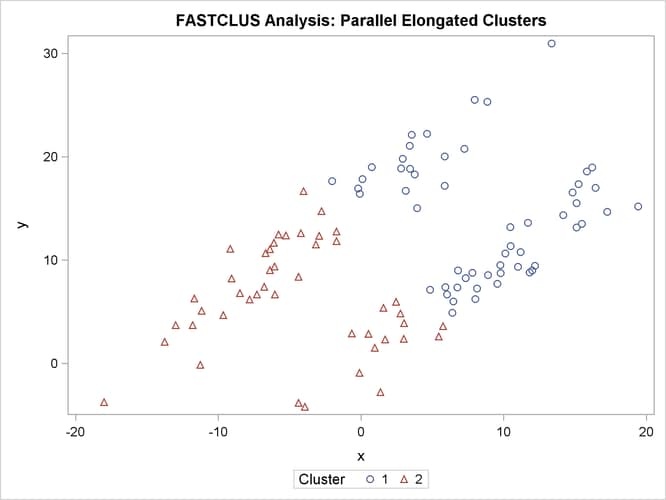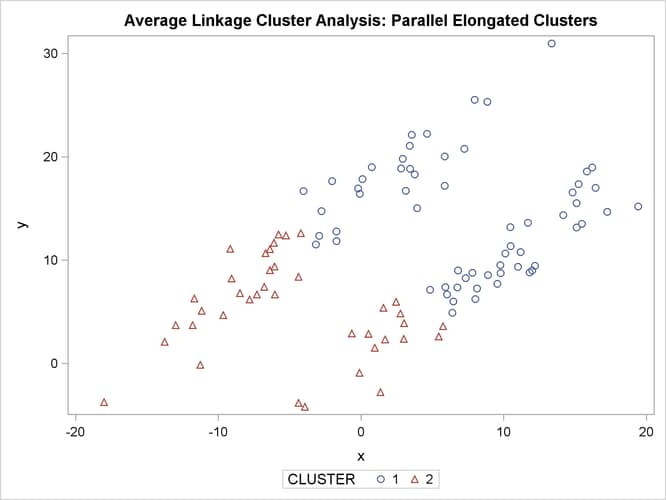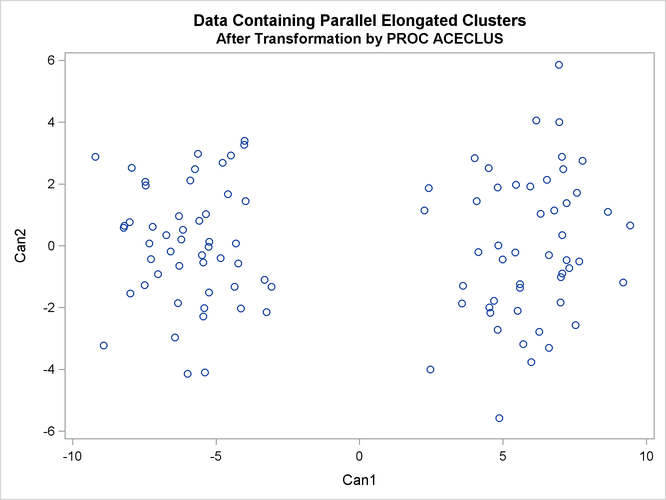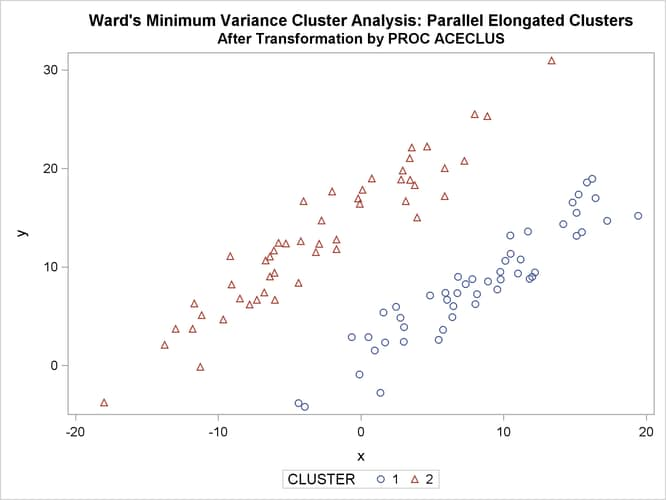### Elongated Multinormal Clusters

In this example, the data are sampled from two highly elongated multinormal distributions with equal covariance matrices. The following SAS statements produce Figure 11.18:

```data elongate;
keep x y;
stop;
generate:
do i=1 to 50;
a=rannor(7)*6+ma;
b=rannor(7)+mb;
x=a-b;
y=a+b;
output;
end;
return;
run;
```
```proc fastclus data=elongate out=out maxc=2 noprint;
run;

%modstyle(name=ClusterStyle2,parent=Statistical,type=CLM,
markers=Circle Triangle circlefilled);
ods listing style=ClusterStyle2;

proc sgplot;
scatter y=y x=x / group=cluster;
title 'FASTCLUS Analysis: Parallel Elongated Clusters';
run;
```

Notice that PROC FASTCLUS found two clusters, as requested by the MAXC= option. However, it attempted to form spherical clusters, which are obviously inappropriate for these data.

Figure 11.18: Parallel Elongated Clusters: PROC FASTCLUSThe following SAS statements produce Figure 11.19:

```proc cluster data=elongate outtree=tree method=average noprint;
run;

proc tree noprint out=out n=2 dock=5;
copy x y;
run;

proc sgplot;
scatter y=y x=x / group=cluster;
title 'Average Linkage Cluster Analysis: '
'Parallel Elongated Clusters';
run;
```

Figure 11.19: Parallel Elongated Clusters: PROC CLUSTER METHOD=AVERAGEThe following SAS statements produce Figure 11.20:

```proc cluster data=elongate outtree=tree method=twostage k=10 noprint;
run;

proc tree noprint out=out n=2;
copy x y;
run;

proc sgplot;
scatter y=y x=x / group=cluster;
title 'Two-Stage Density Linkage Cluster Analysis: '
'Parallel Elongated Clusters';
run;
```

Figure 11.20: Parallel Elongated Clusters: PROC CLUSTER METHOD=TWOSTAGEPROC FASTCLUS and average linkage fail miserably. Ward’s method and the centroid method (not shown) produce almost the same results. Two-stage density linkage, however, recovers the correct clusters. Single linkage (not shown) finds the same clusters as two-stage density linkage except for some outliers.

In this example, the population clusters have equal covariance matrices. If the within-cluster covariances are known, the data can be transformed to make the clusters spherical so that any of the clustering methods can find the correct clusters. But when you are doing a cluster analysis, you do not know what the true clusters are, so you cannot calculate the within-cluster covariance matrix. Nevertheless, it is sometimes possible to estimate the within-cluster covariance matrix without knowing the cluster membership or even the number of clusters, using an approach invented by Art, Gnanadesikan, and Kettenring (1982). A method for obtaining such an estimate is available in the ACECLUS procedure.

In the following analysis, PROC ACECLUS transforms the variables `X` and `Y` into the canonical variables `Can1` and `Can2`. The latter are plotted and then used in a cluster analysis by Ward’s method. The clusters are then plotted with the original variables `X` and `Y`.

The following SAS statements produce Figure 11.21 and Figure 11.22:

```proc aceclus data=elongate out=ace p=.1;
var x y;
title 'ACECLUS Analysis: Parallel Elongated Clusters';
run;

proc sgplot;
scatter y=can2 x=can1;
title 'Data Containing Parallel Elongated Clusters';
title2 'After Transformation by PROC ACECLUS';
run;
```

Figure 11.21: Parallel Elongated Clusters: PROC ACECLUS

 ACECLUS Analysis: Parallel Elongated Clusters

The ACECLUS Procedure

Approximate Covariance Estimation for Cluster Analysis

 Observations Proportion 100 0.1 2 0.001

Means and Standard Deviations
Variable Mean Standard
Deviation
x 2.6406 8.3494
y 10.6488 6.8420

COV: Total Sample Covariances
x y
x 69.71314819 24.24268934
y 24.24268934 46.81324861

Initial Within-Cluster Covariance Estimate = Full Covariance Matrix

 Threshold = 0.328478

Iteration History
Iteration RMS
Distance
Distance
Cutoff
Pairs
Within
Cutoff
Convergence
Measure
1 2.000 0.657 672.0 0.673685
2 9.382 3.082 716.0 0.006963
3 9.339 3.068 760.0 0.008362
4 9.437 3.100 824.0 0.009656
5 9.359 3.074 889.0 0.010269
6 9.267 3.044 955.0 0.011276
7 9.208 3.025 999.0 0.009230
8 9.230 3.032 1052.0 0.011394
9 9.226 3.030 1091.0 0.007924
10 9.173 3.013 1121.0 0.007993

 WARNING: Iteration limit exceeded.

ACE: Approximate Covariance Estimate
Within Clusters
x y
x 9.299329632 8.215362614
y 8.215362614 8.937753936

Eigenvalues of Inv(ACE)*(COV-ACE)
Eigenvalue Difference Proportion Cumulative
1 36.7091 33.1672 0.9120 0.9120
2 3.5420   0.0880 1.0000

Eigenvectors (Raw Canonical
Coefficients)
Can1 Can2
x -.748392 0.109547
y 0.736349 0.230272

Standardized Canonical
Coefficients
Can1 Can2
x -6.24866 0.91466
y 5.03812 1.57553

Figure 11.22: Parallel Elongated Clusters after Transformation by PROC ACECLUSThe following SAS statements produce Figure 11.23:

```proc cluster data=ace outtree=tree method=ward noprint;
var can1 can2;
copy x y;
run;

proc tree noprint out=out n=2;
copy x y;
run;

proc sgplot;
scatter y=y x=x / group=cluster;
title 'Ward''s Minimum Variance Cluster Analysis: '
'Parallel Elongated Clusters';
title2 'After Transformation by PROC ACECLUS';
run;
```

Figure 11.23: Transformed Data Containing Parallel Elongated Clusters: PROC CLUSTER METHOD=WARD